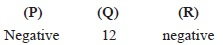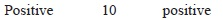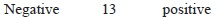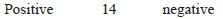# User Forum

Subject :IMO    Class : Class 6

Fill in the blanks.
(i) When two negative integers are added, we get a__(P)__ integer.
(ii) The number of integers between –16 and –2 is __(Q)__.
(iii) When two __(R)__ integers are added, we get a positive integer.

ABCDANSWER SHOULD BE C. BUT, IN SOLUTION, ANSWER FOR Q IS 14. IT IS 13 AS PER SEEN IN NUMBERLINE.